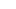# Limitation of Vlookup and How to overcome it by Using Index and Match Function (Upskill Tutorial on Excel)

January 30, 2019

Vlookup as you know is an amazing formula which looks up for a search keyword in a table and returns the desired value from a particular column. But the catch here is that the column which contains your search keyword should be the 1st column in the table and it can return values only from the right columns.

To demonstrate this let's take an example of the below table which can be considered as master database.

Now using the above excel sheet you are asked to find the City for any 2 employee ids suppose you are given the below task:

So the task is to get the name of the city in cell B11 then a vlookup can be used to get the name of the city.

The above formula would give the desired result which would look like the below.

Now Imagine that we only know the Employee Name of some folks but we need their IDs. So a task like the below.

Now vlookup cannot be used here because the emp name "Sam" which you would be searching for falls in the 2nd column of the master table and you can get anything on the right of it, i.e. you can get city name but not Emp ID.

One easy way is to redesign your master table by adding "Emp ID" again on the right and using vlookup.

Suppose the master table is changed to something like this.

Now the task of getting Emp ID is simple using Vlookup.

This will result into below:

This solves the problem to an extent but rarely you will have a choice to copy the columns on the right in the master table.

So another solution to this is using a combination of Index and Match function. To see how it works let's understand how individually Index and Match works.

Considering the below master table.

The Index formula gives the intersection of row and column. So in the above table if I want to know the intersection of 4th row and 2nd column, Index formula would return "Sam". This is assuming that I am selecting the headers as well.

So the formula if written in cell A15 would look like this.

And this would result when hit enter like the below.

So Index takes 3 inputs: 1) Table Array 2)Row number 3) Column number.

Now comes Match Function. Match function in simple terms returns the position of an item in a row/column.

Consider the same master table and let's try to find the position of "Sam" in column "B".

So the formula using "Match" would look like the below in cell A16

Match takes 3 inputs :1) Lookup Value 2) Lookup Array 3) Exact/Approximate match

And this would return 4 as output because "Sam" is in the 4th position in the column starting from B1.

Now using Index and Match we can get the Emp ID as below.

So here we are first writing the index function which takes 3 inputs

1. Table Array : A1:D5

2. Row Number: Now this has to be identified based on the Emp Name so we use match function

1. Match in turn takes 3 inputs

1. Lookup Value: A11

2. Lookup Array: Column where Emp Name is found i.e., B1:B5

3. Exact/Approximate Match: Always Exact Match so 0

3. Column Number: We need Emp ID and we know that its in 1st column in the master table so 1.

And thus this will result in the below table:

This is how we use Index and Match combination instead of Vlookup.

This is one of the examples you learn while taking our class on Advance Excel. For more detailed tutorial and learning contact us to join our classroom or online training.

Whatsapp us at 9985130004 or send an email today to info@upskilltechnologies.com

###### Featured PostsExcel Demo Video

November 14, 2014

1/2